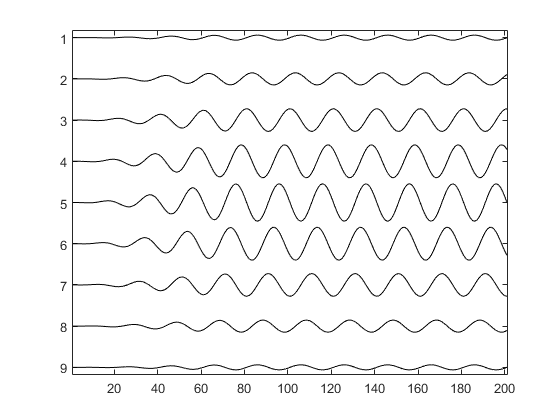createCWSignals

Generate array of CW signals from amplitude and phase.

Syntax

cw_signal = createCWSignal(t_array, freq, amp, phase)
cw_signal = createCWSignal(t_array, freq, amp, phase, ramp_length)

Description

createCWSignals generates a series of continuous wave (CW) signals based on the 1D or 2D input matrices amp and phase, where each signal is given by:

amp(i, j) .* sin(2 .* pi .* freq .* t_array + phase(i, j));
To avoid startup transients, a cosine tapered up-ramp is applied to the beginning of the signal. By default, the length of this ramp is four periods of the wave. The up-ramp can be turned off by setting the ramp_length to 0.

Example:

% define sampling parameters
f = 5e6;
T = 1/f;
Fs = 100e6;
dt = 1/Fs;
t_array = 0:dt:10*T;

% define amplitude and phase
amp = getWin(9, 'Gaussian');
phase = linspace(0, 2*pi, 9).';

% create signals and plot
cw_signal = createCWSignals(t_array, f, amp, phase);
stackedPlot(cw_signal);Inputs

 t_array 1D vector of time points [s] freq frequency of the CW signal [Hz] amp 1D or 2D matrix of amplitudes [au] phase 1D or 2D matrix of phases [rad]

Optional Inputs

 ramp_length length of the up-ramp used to reduce start-up transients in periods (default = 4)

Outputs

 cw_signal matrix of CW signals